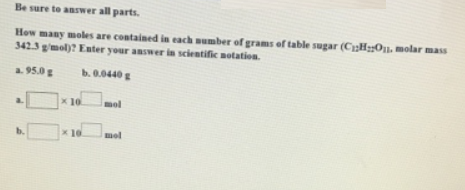# Problem: Be sure to answer all parts.How many moles are contained in each number of grams of table sugar (C 12H22O11 molar mass 342.3 g/mol)? Enter your answer in scientific notation. a. 95.0 g b. 0.0440 g

🤓 Based on our data, we think this question is relevant for Professor Sadeghi's class at UTSA.

###### FREE Expert Solution###### Problem Details
Be sure to answer all parts.
How many moles are contained in each number of grams of table sugar (C 12H22O11 molar mass 342.3 g/mol)? Enter your answer in scientific notation.

a. 95.0 g

b. 0.0440 g